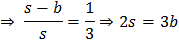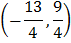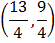# In a, if, then a)are in AP b)are in GP c)are in AP d)are in AP

## Question ID - 51834 :- In a, if, then a)are in AP b)are in GP c)are in AP d)are in AP

3537

(d)

Here,Next Question :
 The verticesof a triangle are (2, 1), (5, 2) and (3, 4) respectively. Then, the circumcentre is a)b)c)d)